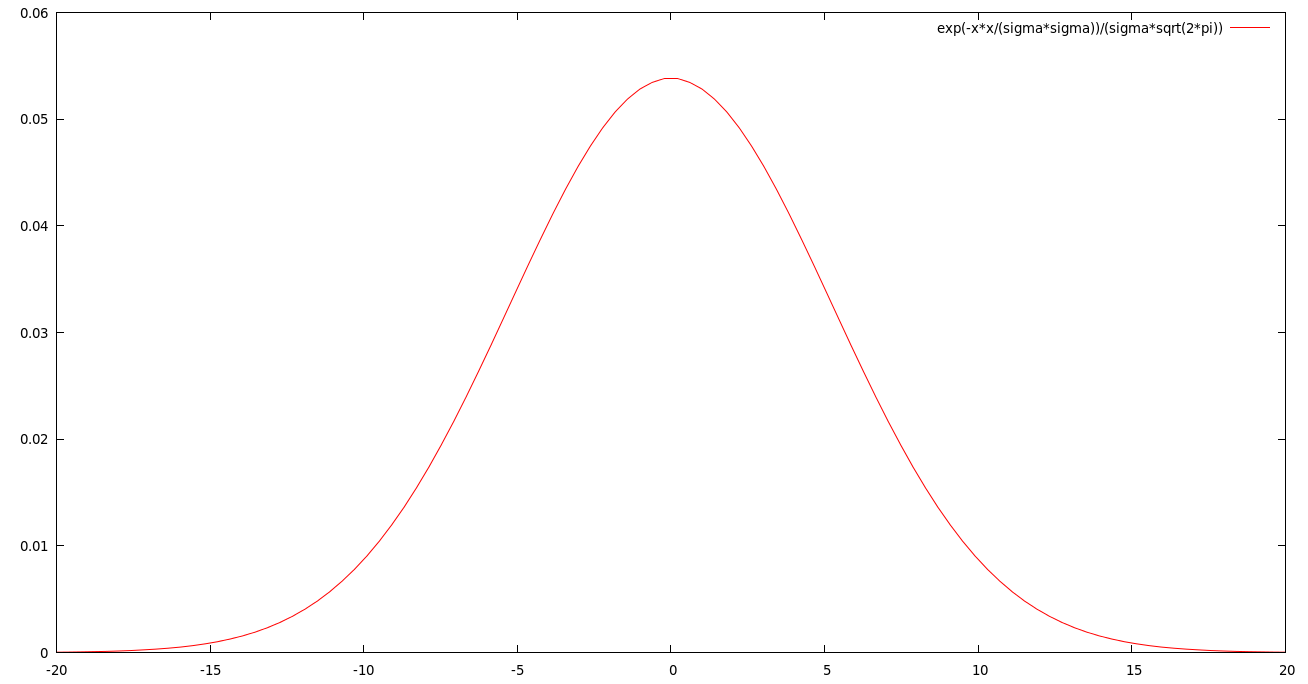Pong

## May 26th 2015, GPS Signal Distribution

With the GPS accuracy describing a confidence interval of 50%, the coordinates of a GPS measurement $m_i$ of a non-moving receiver should be distributed normally under the Gaussian distribution function $\Phi(x)$.

Not exactly. Since we are in 3D space, the coordinates are not statistically independent, so the distribution is not Gaussian, but rather circular Gaussian (or Rayleigh-distributed). Still not exactly. In experiments the real-world distribution shows to be log-normal (see here for a comparison).

For our purposes, however, we project the distribution onto a vertical plane parallel to the trajectory of the receiver. In this case the projections can be considered to be distributed normally on the trajectory plane. For a variance of $\sigma$ and an estimated mean of $\mu=0$ the Gaussian normal distribution is

$\Phi(x) = \frac{1}{\sigma\sqrt{2\pi}}e^{-x^2/2\sigma^2}$

A confidence interval of 50% for a particular accuracy $a$ means that

$P(|x-\mu| \le a) = erf(\frac{ a }{\sqrt{2}\sigma}) = 0.5$

For a given accuracy $a$, the variance $\sigma$ of the corresponding probability distribution is

$\sigma = a \cdot c$ with $c = \frac{1}{erf^{-1}(0.5) \cdot \sqrt{2}} \approx 1.4826$

So the distribution function of the GPS measurements is the following:

$\Phi(x) = \frac{1}{\sigma\sqrt{2\pi}}e^{-x^2/2\sigma^2}$, $\sigma = a \cdot 1.4826$

Here is an example distribution for $a = 5m$ accuracy projected on the trajectory plane (and plotted with gnuplot):

gnuplot> sigma = 5 * 1.4826
gnuplot> plot [-20:20] exp(-x*x/(sigma*sigma))/(sigma*sqrt(2*pi))